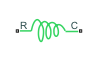# Nonlinear Rotational Spring

Torsional spring based on polynomial or table lookup parameterizations

## Library

Simscape / Driveline / Couplings & Drives / Springs & Dampers

•## Description

The block represents a torsional spring with nonlinear torque-displacement curve. The spring torque magnitude is a general function of displacement. It need not satisfy Hooke’s law. Polynomial and table lookup parameterizations provide two ways to specify the torque-displacement relationship. The spring torque can be symmetric or asymmetric with respect to zero deformation.

The symmetric polynomial parameterization defines spring torque according to the expression:

`$T={k}_{1}\theta +sign\left(\theta \right)\cdot {k}_{2}{\theta }^{2}+{k}_{3}{\theta }^{3}+sign\left(\theta \right)\cdot {k}_{4}{\theta }^{4}+{k}_{5}{\theta }^{5},$`

where:

• T — Spring force

• k1, k2, ...,k5 — Spring coefficients

• θ — Relative displacement between ports R and C, $\theta ={\theta }_{init}+{\theta }_{R}-{\theta }_{C}$

• θinit — Initial spring deformation

• θR — Absolute angular position of port R

• θC — Absolute angular position of port C

At simulation start (t=0), θR and θC are zero, making θ equal to θinit.

To avoid zero-crossings that slow simulation, eliminate the sign function from the polynomial expression by specifying an odd polynomial (b2,b4 = 0).

The two-sided polynomial parameterization defines spring torque according to the expression:

`$T=\left\{\begin{array}{cc}{k}_{1t}\theta +{k}_{2t}{\theta }^{2}+{k}_{3t}{\theta }^{3}+{k}_{4t}{\theta }^{4}+{k}_{5t}{\theta }^{5},& \theta \ge 0\\ {k}_{1c}\theta -{k}_{2c}{\theta }^{2}+{k}_{3c}{\theta }^{3}-{k}_{4c}{\theta }^{4}+{k}_{5c}{\theta }^{5},& \theta <0\end{array},$`

where:

• k1t, k2t, ..., k5t — Spring tension coefficients

• k1c, k2c, ..., k5c — Spring compression coefficients

Both polynomial parameterizations use a fifth-order polynomial expression. To use a lower-order polynomial, set the unneeded higher-order coefficients to zero. To use a higher-order polynomial, fit to a lower-order polynomial or use the table lookup parameterization.

The table lookup parameterization defines spring torque based on a set of torque and angular velocity vectors. If not specified, the block automatically adds a data point at the origin (zero angular velocity and zero torque).

### Variables

To set the priority and initial target values for the block variables prior to simulation, use the Initial Targets section in the block dialog box or Property Inspector. For more information, see Set Priority and Initial Target for Block Variables.

Nominal values provide a way to specify the expected magnitude of a variable in a model. Using system scaling based on nominal values increases the simulation robustness. Nominal values can come from different sources, one of which is the Nominal Values section in the block dialog box or Property Inspector. For more information, see Modify Nominal Values for a Block Variable.

## Ports

`C`

Rotational conserving port

`R`

Rotational conserving port

## Parameters

Parameterization

Select spring parameterization. Options are ```By polynomial``` and ```By table lookup```.

## Version History

Introduced in R2013a